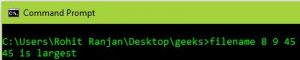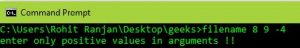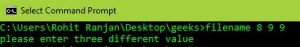# Command line arguments example in C

Prerequisite: Command_line_argument.

The problem is to find the largest integers among three using command line argument.

Notes:

• Command-line arguments are given after the name of the program in command-line shell of Operating Systems. To pass command line arguments, we typically define main() with two arguments : first argument is the number of command line arguments and second is list of command-line arguments.
`int main(int argc, char *argv[]) { /* ... */ }`
• atoi – Used to convert string number to integers

• Examples:

```Input  : filename 8 9 45
Output : 45 is largest

Input  : filename 8 9 9
Output : Two equal number entered

Input  : filename 8 -9 9
Output : negative number entered
```

## Recommended: Please try your approach on {IDE} first, before moving on to the solution.

During execution of program, we pass three integers along with filename of the program and then we will find the largest numbers among three.
Approach :

1. The program “return 1” if any of the two conditions satisfy:
• If any two numbers are same, print the statement “two equal numbers entered”.
• If any number is negative, print “negative number entered”.
2. Else “return 0” if three different integers are entered.

For better understanding run this code on your linux machine.

 `// C program for finding the largest integer ` `// among three numbers using command line arguments ` `#include ` ` `  `// Taking argument as command line ` `int` `main(``int` `argc, ``char` `*argv[])  ` `{ ` `    ``int` `a, b, c; ` ` `  `    ``// Checking if number of argument is ` `    ``// equal to 4 or not. ` `    ``if` `(argc < 4 || argc > 5)  ` `    ``{ ` `        ``printf``(``"enter 4 arguments only eg.\"filename arg1 arg2 arg3!!\""``); ` `        ``return` `0; ` `    ``} ` ` `  `    ``// Converting string type to integer type ` `    ``// using function "atoi( argument)"  ` `    ``a = ``atoi``(argv);  ` `    ``b = ``atoi``(argv); ` `    ``c = ``atoi``(argv); ` ` `  `    ``// Checking if all the numbers are positive of not ` `    ``if` `(a < 0 || b < 0 || c < 0)  ` `    ``{ ` `        ``printf``(``"enter only positive values in arguments !!"``); ` `        ``return` `1; ` `    ``} ` ` `  `    ``// Checking if all the numbers are different or not ` `    ``if` `(!(a != b && b != c && a != c))  ` `    ``{ ` `        ``printf``(``"please enter three different value "``); ` `        ``return` `1; ` `    ``} ` `    ``else` `    ``{ ` `        ``// Checking condition for "a" to be largest ` `        ``if` `(a > b && a > c)              ` `            ``printf``(``"%d is largest"``, a); ` ` `  `        ``// Checking condition for "b" to be largest     ` `        ``else` `if` `(b > c && b > a)  ` `            ``printf` `(``"%d is largest"``, b); ` ` `  `        ``// Checking condition for "c" to be largest.. ` `        ``else` `if` `(c > a && c > b)  ` `            ``printf``(``"%d is largest "``,c); ` `    ``} ` `    ``return` `0; ` `} `

Output :My Personal Notes arrow_drop_upCheck out this Author's contributed articles.

If you like GeeksforGeeks and would like to contribute, you can also write an article using contribute.geeksforgeeks.org or mail your article to contribute@geeksforgeeks.org. See your article appearing on the GeeksforGeeks main page and help other Geeks.

Please Improve this article if you find anything incorrect by clicking on the "Improve Article" button below.

Article Tags :
Practice Tags :

1

Please write to us at contribute@geeksforgeeks.org to report any issue with the above content.